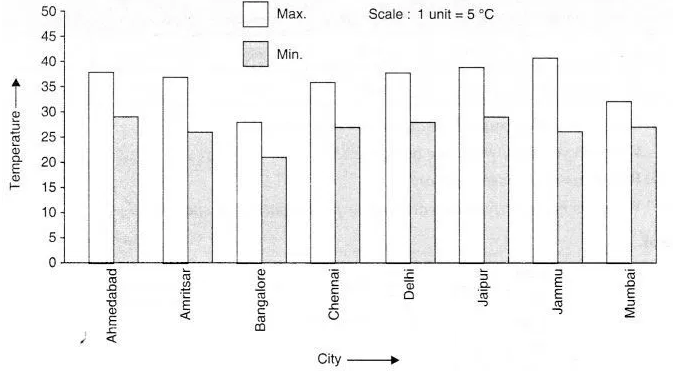# Take the data giving the minimum and the maximum temperature of various cities given in the

Take the data giving the minimum and the maximum temperature of various cities given in the beginning of this chapter. Plot a double bar graph using the data and answer the following:

1. Which city has the largest difference in the minimum and maximum temperature on the given date? .
2. Which is the hottest city and which is the coldest city?
3. Name two cities where maximum temperature of one was less than the minimum temperature of the other.
4. Name the city which has the least difference between its minimum and the maximum temperature.